# Texas Go Math Grade 3 Lesson 1.1 Answer Key Numbers Through Thousands

Refer to our Texas Go Math Grade 3 Answer Key Pdf to score good marks in the exams. Test yourself by practicing the problems from Texas Go Math Grade 3 Lesson 1.1 Answer Key Numbers Through Thousands.

## Texas Go Math Grade 3 Lesson 1.1 Answer Key Numbers Through Thousands

Essential Question
How con you represent 14digit numbers in different ways?

Unlock the problem

The ABC Blocks factory uses boxes of 100 blocks to pack a crate of 1,000 blocks. how many boxes of 100 blocks are in each crate of 1,000?

• Underline what the problem is asking you to find.
• Circle the number you will count by to find the answer.

Count by hundreds to find the total number of boxes of 100 blocks that will go into each crate. Then count the crates.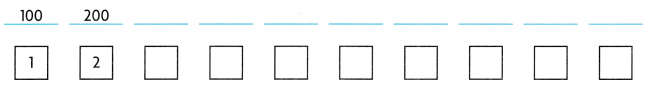So, there are 10 boxes of 100 blocks in each crate of 1,000.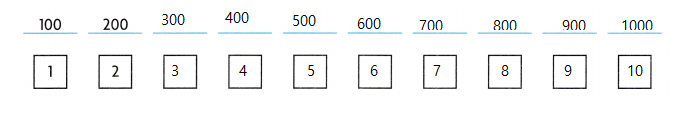Example
ABC Blocks has 2,600 blocks to pack. Suppose the factory has no crates. How many boxes of l00 will it pack?
You know there are 10 boxes of 100 in 1,000, so there are ___ boxes of 100 in 2,000. There are ___ boxes of 100 in 600. Add the boxes. 20 + 6 = ___ So, the factory will pack ___ boxes of 100.

There are 20 boxes in 2000.

There are 6 boxes in 600

20+6 = 26 boxes

So, the factory will pack 26 boxes of 100.

Math Idea
1 crate = 1,000 blocks
1 box = 100 blocks
1 stack = 10 blocks

Math Talk
Mathematical Processes
What if the factory had crates of 1,000 and stacks of 10, but no boxes of 100? Explain how it could pack the blocks.

2,600 can be packed as:

Number of crates =

2000/1000 = 2

Number of stacks =

600/10

= 60

Therefore, the blocks are packed as 2 crates and 60 stacks.

Share and Show

Question 1.
When George packs blocks at ABC Blocks, he likes to use the fewest packages possible. The blocks George packs are shown below. Complete the chart.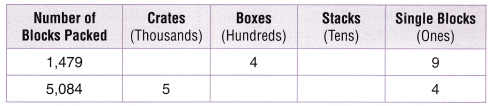Math Talk
Mathematical Processes
Why will there be no boxes for packing 5,084 blocks?

Question 2.
George is packing 1,479 blocks. The factory has no crates or stacks. How can George pack the blocks?

1 crate = 1,000 blocks
1 box = 100 blocks
1 stack = 10 blocks

Without no crates and stacks it is not possible to pack 1,479 blocks using stacks.

Question 3.
A model with base-ten blocks how George can pack 1,479 blocks using the fewest packages. Draw a quick picture to show your model. Then complete the information about the blocks.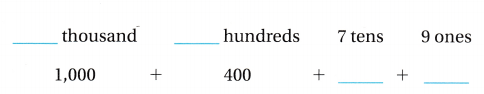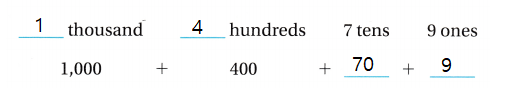Problem Solving

Question 4.
The block factory is packing 2,140 blocks. How can it pack the blocks using the fewest packages? Remember

1 crate = 1,000 blocks
1 box = 100 blocks
1 stack = 10 blocks

2,140 Expanded form : 2000+100+40

Number of crates = 2000/1000 = 2

Number of boxes = 100/100 = 1

Number of stacks = 40/10 = 4

Therefore, The block factory can pack the blocks as 2 crates, 1 box and 4 stacks

Remember
1 crate = 1,000 blocks
1 box = 100 blocks
1 stack = 10 blocks

Question 5.
H.O.T. Suppose the block factory has only boxes and stacks. How can it pack 2,140 blocks?

2140 can be expanded as 2000+100+40

1 box = 100 blocks

2100 /100

= 21 boxes

1 stack = 10 blocks

40/10 = 4 stacks

Now, The block factory can pack 2,140 blocks as 21 boxes and 4 stacks.

Question 6.
Apply Suppose the block factory has only crates and stacks. How can it pack 2,140 blocks?

Total number of blocks = 2,140

2,140 can be expanded as 2000+100+40

1 crate = 1000 blocks

Now, 2000/1000 = 2

1 stack = 10 blocks

140/10 = 14 stacks

Therefore, 2,140 blocks can be packed as 2 crates and 14 stacks.

Unlock the Problem

Question 7.
Multi-Step Janie packs blocks at the ABC Blocks factory. She packs 2 crates, 5 boxes, and 9 stacks. How many blocks did she pack?
(A) 259
(B) 2,509
(C) 2,590
(D) 2,059
Think: 1 crate = 1,000 blocks
1 box = 100 blocks
1 stack = 10 blocks

a. What do you need to find?

We need to find the number of blocks she packed using the given information.

b. What information are you given?

The information given contains the number of crates, boxes and stacks used by Janie to pack the blocks.

c. Draw a quick picture to show the number of thousands, hundreds, and tens. Usefor thousands,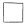for hundreds, and | for tens.

1 crate = 1,000 blocks
1 box = 100 blocks
1 stack = 10 blocks

2 crates, 5 boxes, and 9 stacks.

2 crates = 2 x 1000 = 2000 blocks

5 boxes = 5 x 100 =500 blocks

9 stakes = 9 x 10 = 90 blocks

Now, 2000+500+90 = 2590 blocks

2590 blocks can be shown as|||||||||

d. Complete the sentences.
There are ___ blocks in 2 crates.
There are ___ blocks in 5 boxes.
There are ___ blocks in 9 stacks.

1 crate = 1000 blocks

2 crates = 2 x 1000 = 2000 blocks

So, There are 2000 blocks in 2 crates.

1 box = 100 blocks

5 boxes = 5 x 100 = 500 blocks

So, There are 500 blocks in 5 boxes.

1 stack =10 blocks

9 stacks = 9 x 10 = 90 blocks

So, There are 90 blocks in 9 stacks.

e. Fill in the bubble for the correct answer choice above.

Answer: The correct answer = 2,590 blocks.

Therefore, The number of blocks Janie packed = 2,590

Question 8.
H.O.T. Dan packs 1,500 blocks. He has no boxes. How can he pack the blocks? Explain.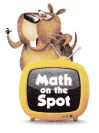1 crate = 1,000 blocks
1 stack = 10 blocks

1500 can be shown as 1000+500

Number of crates = 1000/1000

= 1

Number of stacks = 500/10

= 50

Therefore, Dan can pack the blocks in 1 crate and 50 stakes.

Fill in the bubble for the correct answer choice.

Question 9.
A factory manufactures 100 blocks per hour. How many hours will it take to manufacture 1,300 blocks?
(A) 30 hours
(B) 13 hours
(C) 3 hours
(D) 130 hours

100 blocks = 1 hour

Now, 1300 blocks = 1300/100

= 13

Therefore, the time taken to manufacture 1,300 blocks = 13 hours.

Question 10.
Use Tools Use base-ten blocks to model 2,310. Which model shows the number?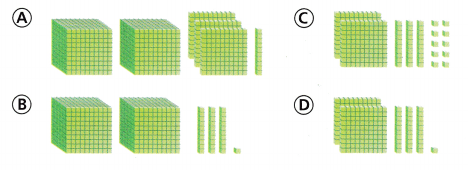2,310 can be shown asQuestion 11.
Multi-Step Ada uses 1,000 beads to make a necklace. She uses 100 beads to make a hair clip. She uses 10 beads to make a ring. Ada used 3,450 beads in all. What could she have made?
(A) 1 necklace, 34 hair clips, and 5 rings
(B) 3 necklaces, 4 hair clips, and 5 rings
(C) 34 necklaces, 5 hair clips, and 0 rings
(D) 3 necklaces, 45 hair clips, and 0 rings

3,450 beads can be shown as

3000+400+50

1000 beads = 1 necklace

100 beads = 1 hair clip

10 beads = 1 Ring

Now,

Number of necklaces =

3000/1000 = 3

Number of hair clips =

400/100 = 4

Number of rings =

50/10 = 5

Therefore, Ada can made 3 necklaces, 4 hair clips, and 5 rings.

Texas Test Prep

Question 12.
Which is NOT a way to pack 1,460 blocks?
(A) 1 crate, 4 boxes, and 6 stacks
(B) 146 stacks
(C) 14 boxes and 6 stacks
(D) 1,460 stacks

1,460 stacks

Explanation:

1 stack=10 blocks

1460 stacks is not equal to 1460 blocks.

### Texas Go Math Grade 3 Lesson 1.1 Homework and Practice Answer Key

Question 1.
Shawna packs blocks at the ABC Blocks factory. She packs thousands in crates and tens in stacks. How can she pack an order for 1,250 blocks using just crates and stacks?

1 crate = 1,000 blocks
1 stack = 10 blocks

Total number of blocks = 1,250

Number of crates =

1000/1000 = 1

Number of stakes =

250/10 = 25

Shawna can his order in 1 crate and 25 stakes.

Question 2.
The block factory has boxes to pack groups of one hundred blocks. If Maks has an order for 2,340 blocks, how can he pack them using the fewest packages?

1 box = 100 blocks
2,340 blocks can be shown in boxes as

2,340/100

= 234

Therefore, the order can be pack in 234 boxes.

Question 3.
Jordy works at the block factory. He has an order to pack 3,280 blocks. How can he pack the blocks using the fewest packages?

Total number of blocks = 3,280

3,280 can be shown as 3000+200+80

1 crate = 1,000 blocks
1 box = 100 blocks
1 stack = 10 blocks

Number of crates =

3000/1000 = 3

Number of boxes =

200/100 = 2

Number of stakes =

80/10 = 8

Therefore, Jordy can pack the blocks in 3 crates, 2 boxes and 8 stakes.

Question 4.
Hakeem has an order for 4,180 blocks to pack, but he has run out of crates to pack the thousands. How can Hakeem pack the blocks?

1 box = 100 blocks
1 stack = 10 blocks

4,180 can be shown as

4000+100+80

Number of boxes =

4000/100 = 40

Also, 100/100 =1

Total : 40+1 = 41 boxes

Number of stakes =

80/10 = 8

Therefore, Hakeem can pack the blocks in 41 boxes and 8 stakes.

Question 5.
Suppose the block factory has only crates and stacks. How can it pack an order for 4,270 blocks?

1 crate = 1,000 blocks
1 stack = 10 blocks

4270 can be shown as

4000+200+70

Number of crates =

4000/1000 = 4

200 can be shown in stakes as 200/10 = 20 stakes

Also, 70/10 =7 stakes

Total stakes = 20+7 = 27

4,270 can be shown as 4 crates, 27 stakes.

Question 6.
Suppose the block factory has only boxes and stacks. How can it pack an order for 3,630 blocks?

1 box = 100 blocks
1 stack = 10 blocks

Now, 3,630 blocks can be shown as

3000+600+30

3000/100 = 30 boxes

600/100 =6 boxes

Total boxes = 30+6 = 36 boxes

30/10 = 3 stakes

Therefore, 3,630 blocks can be packed as 36 boxes, 3 stakes.

Problem Solving

Question 7.
Zack works at the block factory. He needs to pack an order for 1,060 blocks. He only has stacks left to pack with. How many stacks will Zach need?

1 stack = 10 blocks

Total blocks =1060

Now, Number of stakes = 1060/10

= 106

Therefore, Zach need 106 stakes.

Question 8.
Lauren packs an order of blocks in 2 crates and 7 stacks. How many blocks does Lauren pack?

Given that, Lauren packs an order of blocks in 2 crates and 7 stakes.

1 crate = 1,000 blocks

So, 2 crates = 2 x 1000 = 2000
1 stack = 10 blocks

So, 7 stakes = 7 x 10 = 70

Total = 2000+70

=2070

Therefore, Lauren packs 2070 blocks.

Lesson Check

Texas Test Prep

Fill in the bubble completely to show your answer.

Question 1.
Charlene works at a button factory. She packs buttons in boxes of 100. How many boxes will Charlene need to pack 1,500 buttons?
(A) 150
(B) 15
(C) 5
(D) 1,500

15 Boxes

Explanation:

1 box = 100 buttons

1500 buttons

= 1500/100

= 15

Therefore, 15 boxes are needed to pack 1,500 buttons.

Question 10.
A factory fills 10 cups of yogurt every second. How many seconds will it take for the factory to fill 1,800 cups of yogurt?
(A) 18 seconds
(B) 8 seconds
(C) 180 seconds
(D) 1,800 seconds

1 second = 10 cups

180 seconds x 10 cups = 1,800 cups

Therefore, 1800 seconds are required to fill 1800 cups of yogurt.

Question 11.
Darius has 1,026 baseball cards. He wants to use blocks to show the number of thousands, hundreds, tens, and ones. Which shows what Darius can model?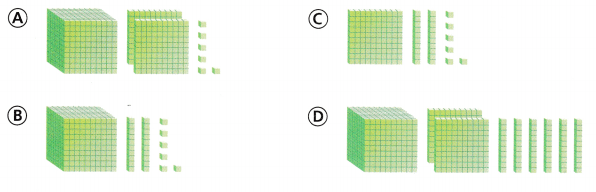This model shows 1,026 baseball cards.

Question 12.
Soo-Lin draws this picture to show the number of pennies she has saved.How many pennies does Soo-Lin have?
(A) 317
(B) 3,170
(C) 3,017
(D) 3,172

3172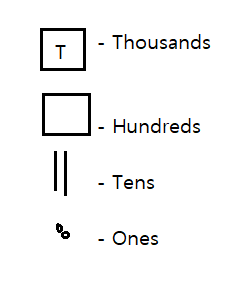Therefore, Soo- Lin have saved 3,172 pennies.

Question 13.
Multi-Step Dennis makes a tile table. He uses 3 boxes of 1,000 red tiles, some boxes of 100 blues tiles, and 8 boxes of 10 green tiles. He uses 3,380 tiles in all. How many boxes of blue tiles does Dennis use?
(A) 13
(B) 30
(C) 130
(D) 300

300 Blue tiles

Explanation:

3 boxes of 1000 red tiles = 3 x 1000 = 3000

8 boxes of 10 green tiles = 8 x 10 = 80

Total = 2000 + 80

= 3080

Total tiles = 3380

Now, Number of blue tiles = 3380 – 3080

= 300

Therefore, Dennis used 300 blue tiles.

Scroll to Top